# Iterated Aut revisited

In an earlier post, I briefly mentioned the idea of repeatedly iterating the Aut operator (a function which takes an abstract group as its input and outputs the automorphism group) and observing the behaviour. There have been papers on the transfinite version of this problem, and a few of the theorems are applicable here. Other results can easily be proved from first principles.

• If Aut(G) is Abelian, then G is cyclic.
• If Aut(G) is cyclic, then |G| is either 1, 2, 4, a power of an odd prime, or twice a power of an odd prime.
• If G is a non-Abelian simple group, then Aut(G) = Aut(Aut(G)).
• If G is centreless, then its automorphism group is also centreless. Moreover, the automorphism tower stabilises in a finite time, resulting in a complete group.

The tree of ancestors of the trivial group is straightforward to compute. It consists entirely of cyclic groups, the orders of which form sequence A117729 in the OEIS. I’ve sketched the first few terms of this tree: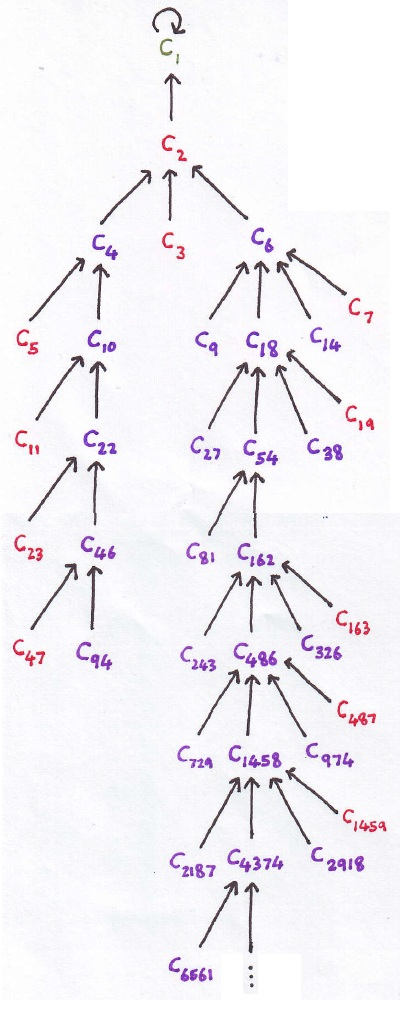Since there are so many groups, I decided to concentrate on observing the behaviour of cyclic groups under iteration of the Aut operator. The automorphism group of the cyclic group of order n is an Abelian group, namely the multiplicative group of totients modulo n. We’ve already covered orders up to and including 7 in the above tree, so let’s see what happens to the cyclic group C8. We first get the Klein 4-group, and then the symmetric group S3: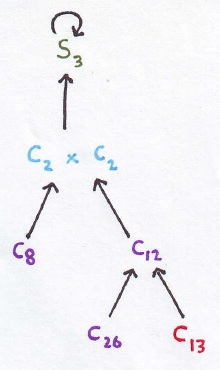In this case, S3 arises as the general linear group over the vector space (F2)². This applies to any direct sum of Cp cyclic groups, such as (F2)³. We get the general linear group GL(3,2), which happens to be isomorphic to PSL(2,7). Its automorphism group is PGL(2,7), which is a complete group (centreless group with no outer automorphisms), and therefore maps to itself.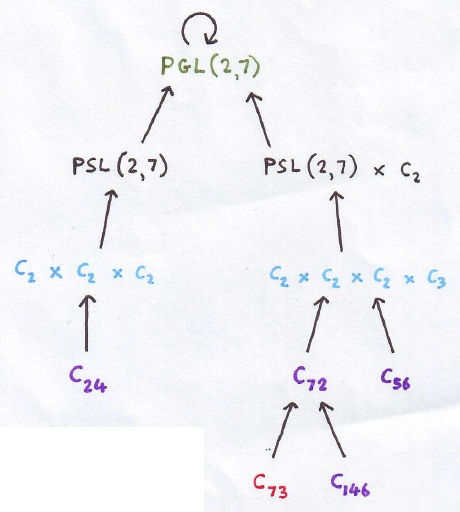So far, all of the ‘terminal groups’ have been complete groups. It turns out that our next cyclic group, C15, will result in a group which is equal to its own automorphism group despite not being centreless. In essence, the existence of a non-trivial centre cancels out the outer automorphism.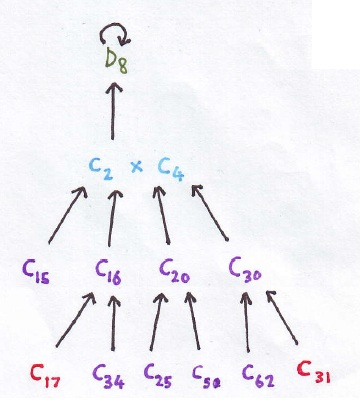In the existing literature, people treat the Aut operator as more than a function on the set of finite groups, and generalise this to a transfinite process. Indeed, they do not consider D8 to have ‘stabilised’, since it is not a complete group, but rather declare that it terminates in ω + 1 steps, with the ωth iterate being the cyclic group C2. It has been proved that these transfinite automorphism towers always terminate. Here, however, we are only interested in isomorphism classes of groups, and finite iterates of the Aut operator, so for our purposes D8 is a terminal group (a group isomorphic to its automorphism group).

The next cyclic group unaccounted for, C21, also stabilises as a dihedral group, namely D12. It is more helpful to visualise this as the direct product of S3 and C2. Again, this is not a complete group, since it is not centreless.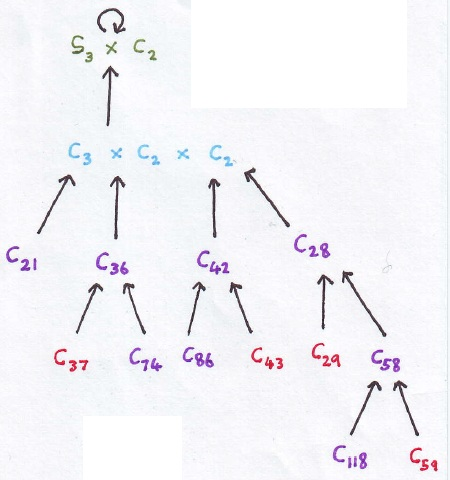Along similar lines to how the general linear group can arise, we also obtain general affine groups. C67, for example, culminates in the direct product of S3 with the general affine group of (F2)², which happens to be S4. This is a direct product of two characteristic groups, so its automorphism group is the product of their automorphism groups. As S3 and S4 are themselves complete, the chain terminates here.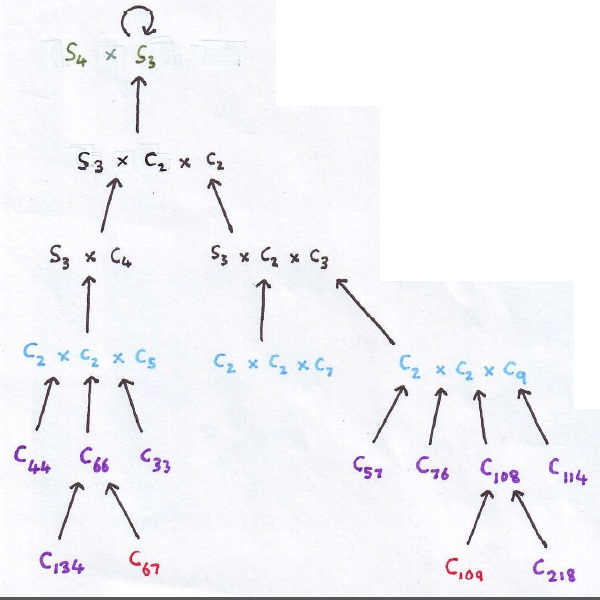### Central and wreath products

As we progress further, computing automorphism groups becomes very difficult. Take the cyclic group C32, for example. Its automorphism group is C2 × C8, and the automorphism group of that can, with some little work, be shown to be C2 × D8. What about the automorphism group of that? It is not too difficult to show that it is the group obtained by placing a lightbulb on each of the vertices of a rectangle, and considering the group generated by Euclidean transformations of the rectangle together with toggling an even number of lightbulbs.

That group has order 32, and is generated by the twelve elements of order 4. Draw an edge between two of these elements if they do not commute with each other; the resulting graph is a pair of disjoint octahedra: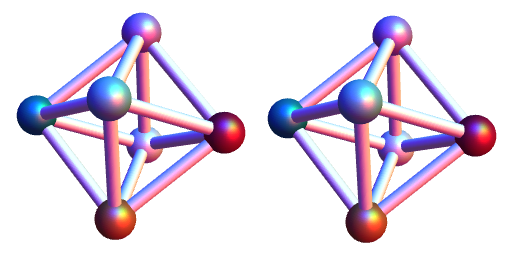So, we have something vaguely similar to a direct product. It transpires that it’s actually the central product of Q8 with itself, obtained from the direct product by quotienting by the common centre (−1, −1). We denote this by Q8 * Q8.

It can be shown that the automorphisms of Q8 * Q8 are products of automorphisms of the individual Q8s, and an automorphism exchanging the two copies of Q8. As the automorphism group of Q8 is the symmetric group S4 (the rotational symmetries of an octahedron), the automorphism group of Q8 * Q8 is the wreath product S4 wr C2. We can think of this as the rigid motions of two identical octahedra; we can rotate them individually or permute them.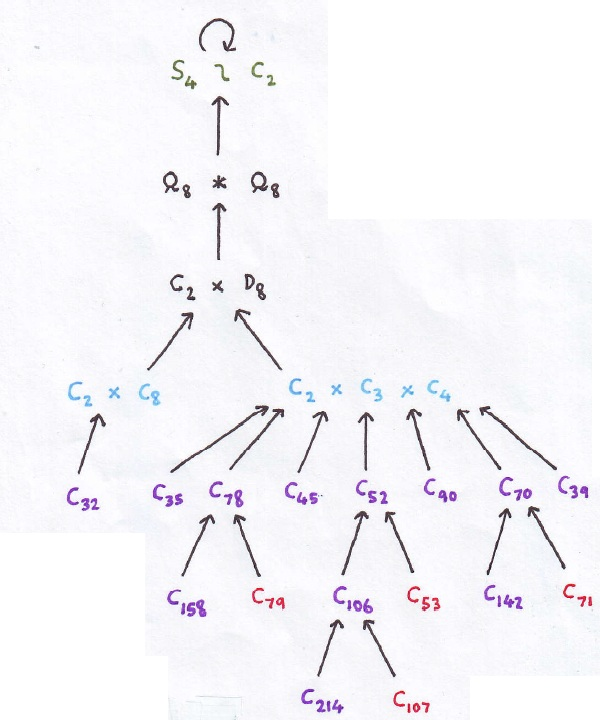I suppose, at this point, I should explain what a wreath product is. I find that it’s rather intuitive to understand, and best explained by considering a few examples. Firstly, if we have a wreath product A wr B, it is necessary for B to act on a set X. We then define A wr B as acting on the set A^X (the set of ways to label the elements of X with elements of A), generated by the action of B permuting the elements of X, and the action of A^X on A^X by pointwise multiplication.

For example, C2 wr Z (where Z is the infinite cyclic group, acting on the integers by translation) is the lamplighter group, generated by translations of an infinite line of lightbulbs and by toggling any subset of the lamps.

For all n other than 2, 3 and 6, we have Aut(An) = Aut(Sn) = Sn. For n = 6, the automorphism group of A6 and S6 is the projective semilinear group PΓL(2, 9), which is twice as large (with order 1440, instead of 720). This is a result of the exceptional outer automorphism of S6.

As a challenge, try tracking the behaviour of the prime cyclic group C227. (Hint: you’ll need to know lots of four-dimensional geometry!) If you give up, it’s included on this beautiful colour-coded poster, along with many other groups.

### Infinite growth?

We conjecture that infinite growth is possible, and that the sequence C61 → C60 → C2 × C2 × C4 → D4 → F4/Z × C2 → (S4 wr C2) × C2 → (S4 wr C2) × C2 × C2 → (S4 wr C2) × S4 × C2 × C2 → (S4 wr C2) × S4 × S4 × C2 × C2 × C2 × C2 → … is such an example. It appears to result in arbitrarily many copies of C2 being produced, thereby expanding infinitely. We haven’t ruled out the possibility that the other groups produced in the process could interfere with this and prevent further growth, and it seems extremely difficult to prove that there exists a group capable of infinite growth.

If infinite growth is possible, it would be unsurprising if it is undecidable whether a generic finite group terminates or grows indefinitely. Indeed, it may even be possible that there exists an algorithmic procedure for converting a Turing machine into a group, which terminates under iteration of Aut if and only if the Turing machine halts. If this is the case, it is probably the most difficult Turing-complete programming language in existence.

This entry was posted in Uncategorized. Bookmark the permalink.

### 9 Responses to Iterated Aut revisited

1.Thomas says:

What is this D4 group?

•apgoucher says:

The Weyl/Coxeter group corresponding to the D4 root lattice. It has order 192, and can be realised as the group of all invertible 4-by-4 matrices containing twelve ‘0’s, an even number of ‘+1’s and an even number of ‘-1’s.

2.Thomas says:

C40 and C41 are smaller examples of cyclic groups that go to D4, so if that diverges, then those would be the smallest cyclic divergent groups

•apgoucher says:

Yes, that’s correct.

•Thomas says:

Also, it seems highly likely that somewhere along the line, a group is produced that interferes with the divergence, because that is exactly what happens with D4: D4 appears to diverge, but D4*C2 goes into a loop pretty quickly

•apgoucher says:

If the general affine groups over F_2 are complete and non-interacting, then no interference occurs.

3.pzqalex says:

According to LMFDB, Aut(C2 x D4) has order 64 instead of 32 (as claimed in the post).